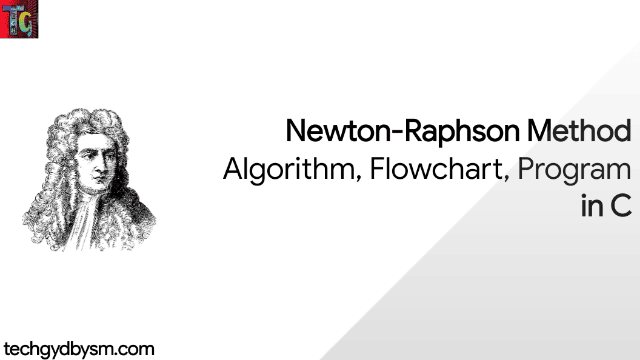# Newton-Rapshon Method: Algortihm, Flowchart, Program in C

C Programs has multiple application in several branches of science. One such application is in Numerical Analysis. The following article will guide you through the algorithm, flowchart and C Program to evaluate a Newton-Raphson Method Numerical Problem using C Language.Newton Raphson Method in C

## What is Newton-Raphson Method?

This is an iterative method and is used to find isolated roots of an equation f(x)=0. The object of this method is to correct the approximate root x0(suppose) successively to its exact value x1. Initially, a crude approximation small interval [a0,b0] is found out in which only one root x1(suppose) of f(x)=0 lies.

## Computation Scheme

Let x=x0 (a0<x0<b0) is an approximation of the root x1 is the equation f(x)=0. Let h be small correction on x0, then x2=x0+h is the correct root.
Thus, f(x1)=0 => f(x0+h)=0.
f(x0) + hf'(x0) + h²f"(x0)/2! + … = 0.
As h is small, neglecting the second and higher power of h, we get,
h = -f(x0)/f'(x0)
Thus, x2 = x1 + h = x1- f(x0)/f'(x0).
Further, if h1 be the correction on x2, then
x3 = x2 + h1 is the correct root of f(x)=0.
Then, f(x3) = f(x2+h1) = 0.Bisection Method: Algortihm, Flowchart, Program in C
So, f(x2) + h1f'(x2) + (h1)²f"(x2)/2! + … = 0.
Neglecting, the second and higher powers of h1, we get,
h1 = -f(x2)/f'(x2).
Thus, x3 = x2 + h1 = x2 - f(x2)/f'(x2).
Proceeding in this manner, we get the (n+1)th corrected root as,
xn+1 = xn - f(xn)/f'(xn).
The formula above generates a sequence of successive correction on an approximate root to get the correct root x1 of f(x)=0, provided the sequence is convergent. This is the iteration formula for Newton-Raphson Method. The number of iteration depends upon the desired degree of accuracy of the root.

## Algorithm

STEP 1: Read x0 (the initial guess) and eps (the allowable error)
STEP 2: Define f(x)
STEP 3: Compute x1=x0-f(x0)/f'(x0) and t = x1-x0.
STEP 4: If |t|>eps, then replace x0 by x1 and goto STEP 3, otherwise print the root is x1 and terminate the Algorithm.

## FlowchartNewton--Raphson Method

## Sample Program

WAP in C to evaluate root of f(x)=3x-cos x -1 using N-R Method.

#### Code:

``````#include<stdio.h>
#include<conio.h>
#include<math.h>                  /*header files included*/
float f(float x)                  /*declaring the function f(x)*/
{
float y;
y=3*x-cos(x)-1;
return y;
}
float f1(float x)                 /*declaring the function f'(x)*/
{
float z;
z=3+sin(x);
return z;
}
void main()
{
int n=0;
float x0,xn,err=1,h;
clrscr();
printf("\nEnter the initial value of x = ");       /*asking for the intial value of x*/
scanf("%f",&x0);
printf("\n n\t xn \t\t f(xn)\t\t f'(xn)\t\tx(n+1)\n");    /*designing the table*/
while(fabs(err)>.000000001)
{
h=-(f(x0)/f1(x0));                    /*working formula*/
xn=x0+h;
err=xn-x0;
printf("\n %d\t %.10f\t %.10f\t %.10f\t %.10f\n",n,x0,f(x0),f1(x0),xn);     /*formatting values in output*/
x0=xn;
n++;
}
printf("\n One real root of the given equation is %.8f (correct upto 8 decimal places)",xn);        /*printing the final answer*/
getch();
}``````

#### Compiler Window:Newton Rapshon Method

#### Output Window:Output Screen

## Video tutorial:

Feel free to comment below if you are facing problems while executing this program. Visit again soon.